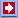Software Online Library ContactsSoftware: Business&GamesCalculator Download Analyzer Mail NavigatorOnline: Stat Calc Y(X) Finder 2D Plotter 3D Plotter Science CalcLibrary: Data Mining Statistics Machine Learning Genetic Algorithms Neuronets Fuzzy logic ForecastLinksContactsOnline -> 3D Plotter

 QuData 3D Plotter This application allows you to build 3D plot of a function dependent on two variables: X and Y. Enter the function in the text field labeled "U = " below the plot and press to draw it. The surface is plotted with the help of square polygons. You can also plot a color map of the surface. Choose the "Map View" to build a colorized map of heights. The regions of maximum height are drawn in red and the low regions are blue. The scale of colors and heights is given on the left of the plot. Your functions can contain: Numbers the decimal part of the number must be separated by a dot. Operations. Following operations are permitted: "+" - add "-" - subtract "*" - multiply "/" - divide "^" - power Functions. Function consists of a name and a set of arguments in parentheses. Arguments are separated by comma (if a function has more than one argument). The following standard functions are available: "sin(x)" - sine; "cos(x)" - cosine; "tan(x)" - tangent; "ctg(x)" - cotangent; "asin(x)" - arc sine; "acos(x)" - arc cosine; "atan(x)" - arc tangent; "sinh(x)" - hyperbolic sine; "cosh(x)" - hyperbolic cosine; "tanh(x)" - hyperbolic tangent; "exp(x)" - ex where e = 2.718...; "ln(x)" - returns the natural logarithm (base e) of x; "log(x)" - returns the logarithm (base 10) of x; "abs(x)" - absolute value of x; "sqrt(x)" - square root of x (x0.5); "pow(x, y)" - returns x raised to the power y; "max(x, y)" - returns maximum value from x and y; "min(x, y)" - returns minimum value from x and y; "random(d)" - returns uniformly distributed random number greater than 0.0 and less than 1.0, for any d; "fact(x)" - returns factorial of x (x is converted into integer); "rint(x)" - returns the integer value closest to x; "ceil(x)" - returns the closest integer value greater than x; "floor(x)" - returns the closest integer value less than x; Variable: Only the variables named x and y are available In the bottom panel you can enter the Rotation and Inclination angles (in degrees) to turn the view of the surface. Also you can change the Inclination angle by drop and drag your mouse in vertical direction. The Rotation angle is changed if you drop and drag your mouse in horizontal direction. Buttons "Zoom In" (equals to single click of the left button of the Mouse inside 3D Plot) and "Zoom Out" (equals to single click of the right button of the Mouse inside 3D Plot) zoom In or Out the view of the surface. In the bottom row you can choose the range of variables and the height of the plot.